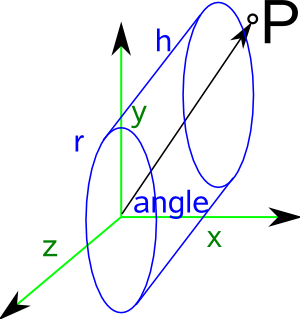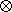# Maths - Changing Coordinates

An important topic for tensors is the way that they are affected by a change in coordinates. Also can we formulate our tensor equations in such a way that they are independent of the chosen coordinate system?

First we will start with a coordinate system based on a linear combination of orthogonal basis vectors.The physical vector 'p' can be represented by either:

p = ∑ viei in the red coordinate system and

p = ∑ v'ie'i in the green coordinate system.

where:

• p = physical vector being represented in tensor terms
• vi = tensor in the red coordinate system
• ei= basis in the red coordinate system
• v'i= tensor in the green coordinate system
• e'i = basis in the green coordinate system

So we can transform between the two using:

∑ v'k = tki v i

or

∑ ek = t'ki e'i

where:

• t = a matrix tensor which rotates the vector v to vector '
• t' = a matrix tensor which rotates the basis e to the basis e'

## Orthogonal Coordinates

We are considering the situation where a vector is measured as a linear combination of a number of basis vectors. We now add an additional condition that the basis vectors are mutually at 90° to each other. In this case we have:

ei • ej = δij

where:

• ei = a unit length basis vector
• ej = another unit length basis vector perpendicular to the first.
• δij= Kronecker Delta as described here.

If we choose a different set of basis vectors, but still perpendicular to each other, say e'i and e'j then we have:

e'i • e'j = δij

To add more dimensions we can use:

eki • ekj = δij

This is derived from the above expression using the substitution property of the Kronecker Delta.

We could express the above in matrix notation:

aT a = [I]

where:

• a = a vector
• aT= transpose of 'a'
• [I] = the identity matrix

We can combine these terminologies to give:

(aT a)ij = eki • ekj = δij

Any of these equations defines an orthogonal transformation.

## Curvilinear Coordinate SystemsThe simplest case contravarient and covarient tensors is to look at contravarient and covarient vectors. These

• The different ways that they vary with a change in coordinates shown by the partial differentials below.
• As duals of each other - for example in 3D vectors and bivectors are duals.
• Represented as column or row vectors.

The tensor product of two vectors ei and ej may be denoted by ei ej or the operation may be shown explicitly by eiej

A tensor can then be represented as:

∑ aij ei ej

 metadata block see also: Correspondence about this page Book Shop - Further reading. Where I can, I have put links to Amazon for books that are relevant to the subject, click on the appropriate country flag to get more details of the book or to buy it from them.Schaum's Outline of Theory and Problems of Tensor Calculus - I'm finding this hard going, it starts off with as review of linear algebra, matrix notation, etc. It redefines a lot of conventions which are hard to relearn, such as superscrips instead of subscripts to identify elements, and a summation convention, then it goes into coordinate transformations. I cant find any definition of what a tensor is. I think this book is aimed at people who already have some knowledge of the subject. Specific to this page here:

This site may have errors. Don't use for critical systems.